# Literary Attention vs. City Population¶

Measure relationship between literary attention as measured by geographic location occurrence count in 1851-1875 U.S. fiction and populations of 23 U.S. cities between 1790 and as late as 1990. There's a fuller write-up on my blog.

## Preliminaries¶

In :
%matplotlib inline

import pandas as pd
pd.set_option('max_columns', 50)

import numpy as np
import matplotlib.pyplot as plt
import seaborn as sns


## Data¶

In :
cities = pd.read_csv('https://gist.githubusercontent.com/wilkens/9d6ea82c3f07fb7aed68/raw/48dc8d25b19a49cde258190ee8cea42a65722c4f/cities_data.csv', index_col=0)

Out:
city year population literature
0 New York 1790 33100 10603
1 Washington 1790 2800 5079
2 Boston 1790 18300 4568
4 New Orleans 1790 5300 1590
In :
cities.describe()

Out:
year population literature
count 483.000000 483.000000 483.000000
mean 1890.000000 800996.687371 1424.000000
std 60.615789 1985015.220347 2340.399277
min 1790.000000 0.000000 112.000000
25% 1840.000000 21300.000000 229.000000
50% 1890.000000 101000.000000 637.000000
75% 1940.000000 623000.000000 1172.000000
max 1990.000000 16754000.000000 10603.000000
In :
# Work with (base 10) log-scale data
cities_log = cities.replace(0,10) # Change zero populations to avoid log problems
cities_log['population'] = cities_log['population'].apply(np.log10)
cities_log['literature'] = cities_log['literature'].apply(np.log10)
c19_log = cities_log[cities_log.year <= 1900]


## Plots and calculations¶

In :
# Plot lit vs. pop by census year for c19
sns.set_context("talk")
figure = sns.lmplot("population", "literature", col="year", hue="year", data=c19_log,
col_wrap=4, ci=95, palette="muted", size=3,
scatter_kws={"s": 50, "alpha": 1})
figure.set(xlim=(0,8), ylim=(1.5,4.5))

Out:
<seaborn.axisgrid.FacetGrid at 0x119c21588>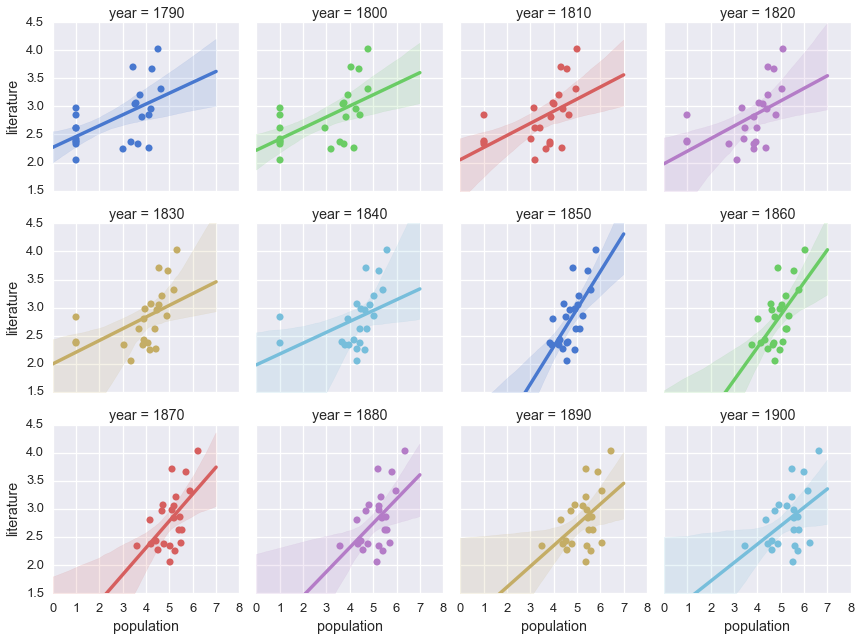In :
# Plot lit vs. pop by census year for all years
figure = sns.lmplot("population", "literature", col="year", hue="year", data=cities_log,
col_wrap=6, ci=95, palette="muted", size=3,
scatter_kws={"s": 50, "alpha": 1})
figure.set(xlim=(0,8), ylim=(1.5,4.5))

Out:
<seaborn.axisgrid.FacetGrid at 0x117ed2438>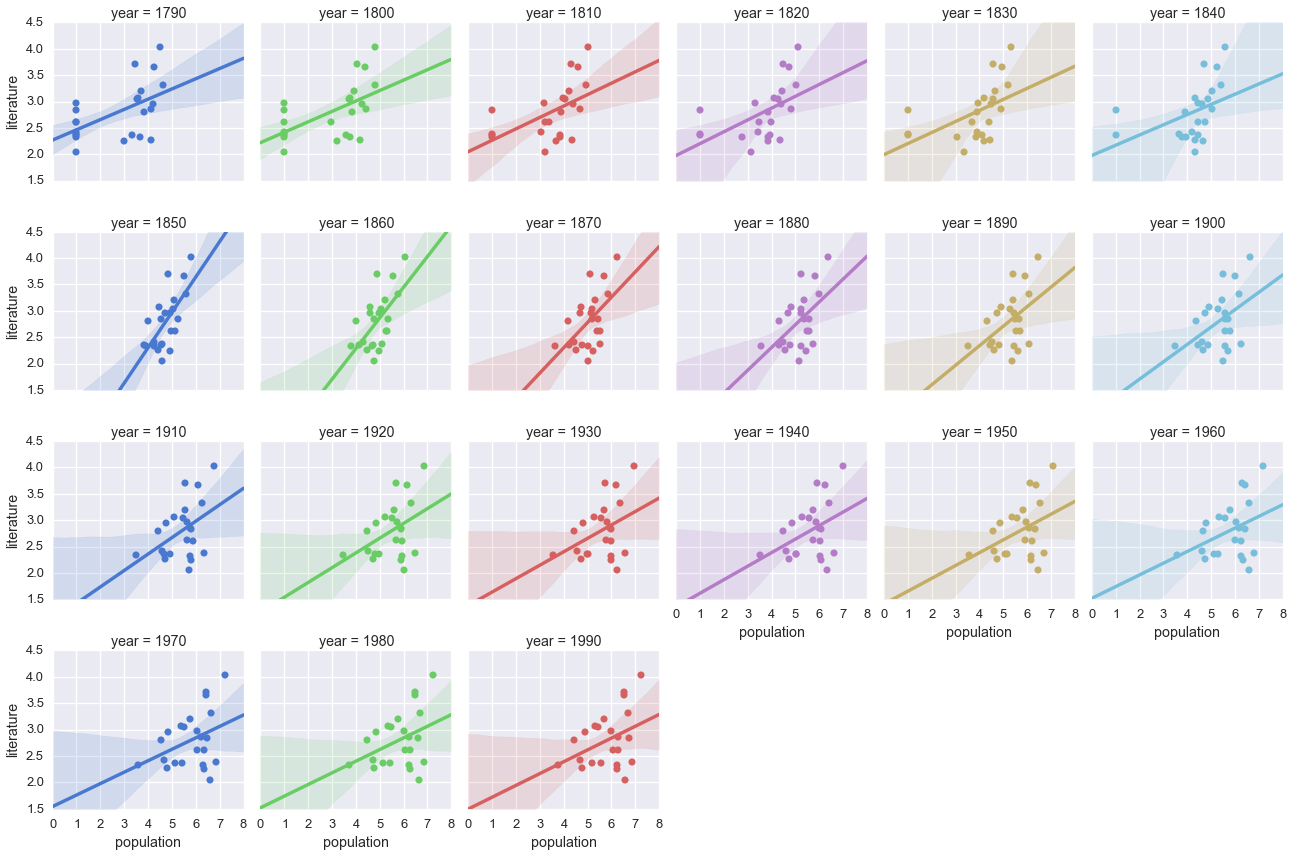OK, this all looks nice, but it's hard to compare the goodness of fit between census years. So let's measure the $r^2$ value for each year, then plot that by year to look for the highest values.

In :
from scipy import stats

cities_na = cities_log.replace(1.0,np.nan) # Get rid of zero-population cities
years = []
rsqs = []
pvals = []
for year in range(1790,2000,10):
years.append(year)
data = cities_na[cities_na.year == year]
pops = data['population']
lits = data['literature']
rsqs.append(r_value**2)
pvals.append(p_value)
# Munge the data back into a frame
data_na = {'year':years, 'Rsq':rsqs, 'p':pvals}
fits_na = pd.DataFrame(data_na)

In :
# Plot the r^2 values
plt.figure(figsize=(8, 6))
plt.scatter(fits_na.year,
fits_na.Rsq,
s=75, c='royalblue')
titlestring = r'$r^2$ vs. Census Year'
plt.title(titlestring)
plt.xlabel('Census Year')
plt.ylabel(r'$r^2$')
plt.xlim(1780,2000)

Out:
(1780, 2000)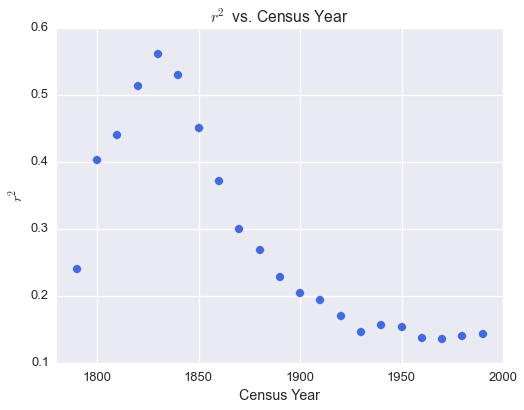In :
# And p values
plt.figure(figsize=(8, 6))
plt.scatter(fits_na.year,
fits_na.p,
s=75, c='royalblue')
titlestring = 'p vs. Census Year'
plt.title(titlestring)
plt.xlabel('Census Year')
plt.ylabel('p')
plt.xlim(1780,2000)

Out:
(1780, 2000)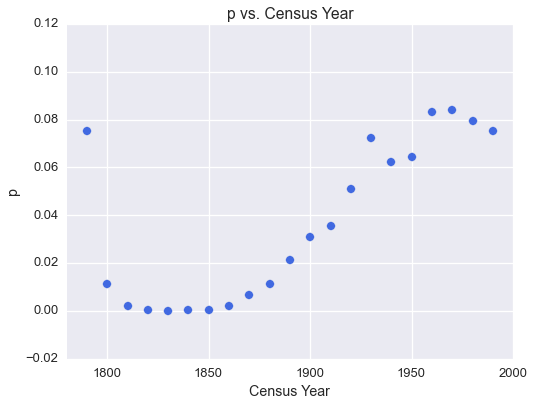In :
# Return to the Rsq values, now with a Gaussian fit
from scipy.optimize import curve_fit
from scipy import asarray as ar,exp

# Define Gaussian function for fitting
def gauss(x,a,x0,sigma):
return a*exp(-(x-x0)**2/(2*sigma**2))

# Constants to normalize the fit
startyear = 1790
baseline = min(fits_na['Rsq']) # Gaussian curve decays to zero, so need to shift the baseline to make it work

x = ar(fits_na['year']-startyear)
y = ar(fits_na['Rsq']-baseline)
mean = x.mean()
sigma = x.std()

# Run the fit
popt, pcov = curve_fit(gauss, x, y, p0=[1,mean,sigma])

# Find x for max(y)
max_val = 0
max_year = 0
for i in range(0,200,1):
j = gauss(i,*popt)
if j > max_val:
max_val = j
max_year = i
print(max_year+startyear, max_val+baseline)

1832 0.535714383789


Note that the highest fitted $r^2$ value occurs in 1832. For comparison, the average book in the dataset was published in 1862.5 and the average location occurred in 1863.2. So almost exactly 30 years lag on average.

In :
# Plot fitted data
plt.figure(figsize=(8, 6))
# Smoother fit line
xs = np.linspace(0,200,400)
plt.plot(xs+startyear, gauss(xs,*popt)+baseline, linewidth=1.0, c='black')
plt.scatter(x+startyear, y+baseline, s=75, c='royalblue')

plt.title('Fit Quality over Time')
plt.xlabel('Census Year')
plt.ylabel(r'$R^2$')
plt.xlim(1780,2000)

Out:
(1780, 2000)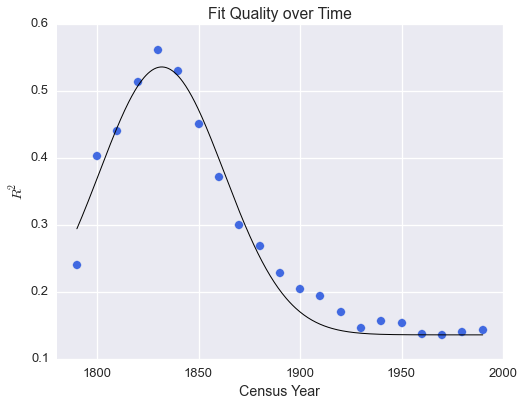## Include zero-population cities¶

For reference, here's what the data would look like if we didn't drop zero-population cities in early years.

In :
# Run a bunch of regressions
from scipy import stats
years = []
rsqs = []
pvals = []
for year in range(1790,2000,10):
years.append(year)
data = cities_log[cities_log.year == year]
pops = data['population']
lits = data['literature']
gradient, intercept, r_value, p_value, std_err = stats.linregress(pops,lits)
rsqs.append(r_value**2)
pvals.append(p_value)
# Munge the data back into a frame
data = {'year':years, 'Rsq':rsqs, 'p':pvals}
fits = pd.DataFrame(data)

In :
# Plot the r^2 values
plt.figure(figsize=(8, 6))
plt.scatter(fits.year,
fits.Rsq,
s=75, c='royalblue')
titlestring = r'$r^2$ vs. Census Year'
plt.title(titlestring)
plt.xlabel('Census Year')
plt.ylabel(r'$R^2$')
plt.xlim(1780,2000)

Out:
(1780, 2000)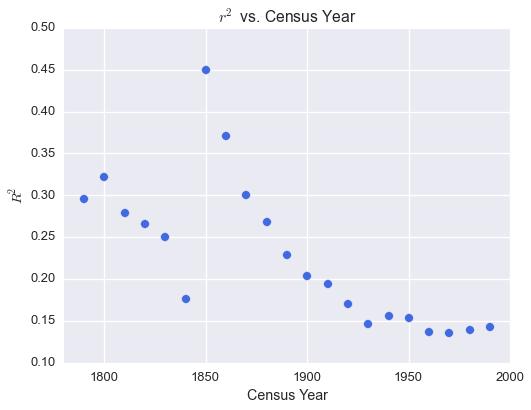In :
# Plot the p values
plt.figure(figsize=(8, 6))
plt.scatter(fits.year,
fits.p,
s=75, c='royalblue')
titlestring = 'p vs. Census Year'
plt.title(titlestring)
plt.xlabel('Census Year')
plt.ylabel('P')
plt.xlim(1780,2000)

Out:
(1780, 2000)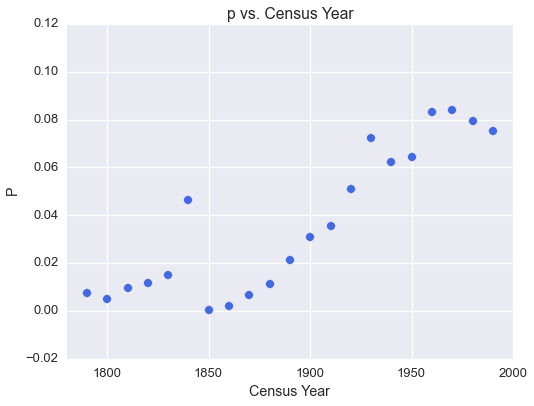In [ ]: